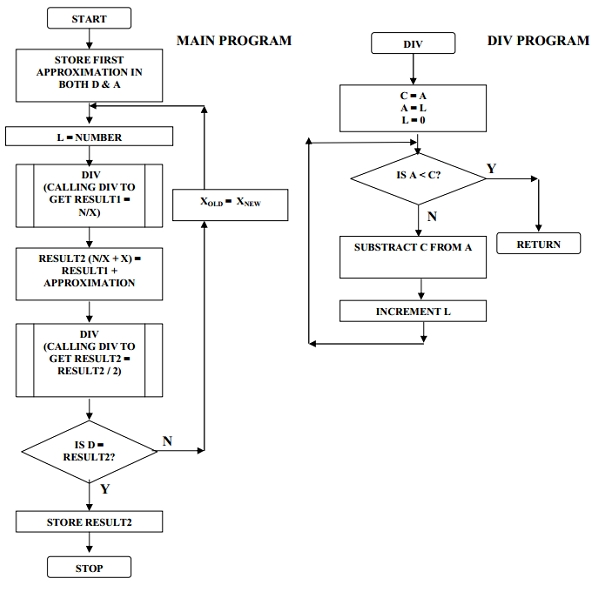# 8085 program to find square root of a number

Now let us see a program of Intel 8085 Microprocessor. This program will find the square root of a number.

### Problem Statement

Write an assembly language program to find the square root of a number in L and store the result at location 8100H

### Discussion

Here we are using division method for finding square root of a number. As we know there is no division operation in 8085, so we are creating division subroutine.

Let N is the number.

X = √N

X2 = N

X2 + X2 = N + X2

2X2 = N + X2

X2 = (N + X2)/2

X =((N + X2)/X)/2

XNew=(N / X + X)/2

If X = XNew then X = √N

### Input

We have tested with three inputs: These inputs are −

• 64H (100D)
• 51H (81D)
• 90H (144D)

### Flow Diagram### Program

HEX Codes
Labels
Mnemonics
8000
80, 00, 83
START
LXI SP, 8300H
SP = 8300H
8003
3E, 01

MVI A, 01H
A (first approximation) = 01 H
8005
57
UP
MOV D, A
D = A Tracking Xold
8006
2E, NUM

MVI L, NUM
L = number N whose square root is to be calculated
8008
CD, 1E, 80

CALL DIV
Call div subroutine to calculate N/X HereN in L and X in A. It performs L = L/A and it returns result in L
800B
7D

MOV A, L
A = L (return argument)
800C
82

A = A+D (N/X) + X
800D
26, 00

MVI H, 00H
H = 00 H
800F
6F

MOV L, A
L = A
8010
3E, 02

MVI A, 02H
A = 02 H
8012
CD, 1E, 80

CALL DIV
Call div subroutine Here sum in L and 2in A Xnew= ((N/Xold)+ Xold)/2
8015
7D

MOV A, L
A = L
8016
BA

CMP D
Compare D and A Comparing Xold and Xnew
8017
C2, 05, 80

JNZ UP
Is A = D, if no go to up If they are equal then the obtained value of X is the answer
801A
32, 00, 81

STA 8100H
Store the result at the desired memory location 8100H
801D
76

HLT
Stop
801E
4F
DIV
MOV C, A
C = A
801F
7D

MOV A, L
A = l
8020
2E, 00

MVI L, 00H
L = 00
8022
B9
UP1
CMP C
Compare a and c registers
8023
D8

RC
8024
91

SUB C
A = A – C
8025
2C

INR L
L = L + 1
8026
C3, 22, 80

JMP UP1
Go back to UP1
8000
80, 00, 83
START
LXI SP, 8300H
SP = 8300H

first output

Data
.
.
.
.
.
.
8100
0A
.
.
.
.
.
.

second output

Data
.
.
.
.
.
.
8100
09
.
.
.
.
.
.

third output

Data
.
.
.
.
.
.
8100
0C
.
.
.
.
.